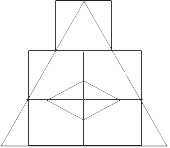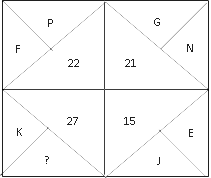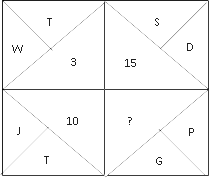# Motorola Reasoning Question

DIRECTIONS for questions 1-3: Seven poles A, B, C, D, E, F and G are put in such a way that the distance between the next two decreases by 1 meter. The distance between the first two poles, A and B is 10 meters. Now answer the following questions ?
1. What is the distance between the first pole A and the last pole G ?
1. 40 m.
2. 49 m.
3. 45 m.
4. None of these.
If we place A,B,C,D,E,F and G sequentially leaving a distance of 10,9,8,7,6,5 respectively the total length comes out to be 45m.
2. If a monkey hops from G to C pole, how much distance did it cover?
1. 26 m.
2. 19 m.
3. 22 m.
4. None of these.
If one pole is removed then a total length of 45 m is distributed equally among 6 pole positions ,hence each pole is placed 9 m away from the other.
1. 8 1/2
2. 7 1/2
3. 9
4. None of these.
All the other three are nouns and EAT is a verb.
DIRECTIONS for questions 4-6: In these questions there are four groups of letters, words or numbers listed under numbers (1) to (4). One of the group does not belong to the same category as others. Find the odd one out.
1. BAT
2. RAT
3. EAT
4. MAT
All the others are in the form of n*n*n − 1 form and 324 is 18*18.
1. 999
2. 3374
3. 2196
4. 324
In all the others first two letters repeat.
1. USTO
2. OOTU
3. TTOU
4. SSTO
No repetition on any letter
DIRECTIONS for questions 7-8: Study the following figure carefully and answer these questions.• How many squares are there in the figure?
1. 5
2. 6
3. 7
4. 8
Obvious from counting.
• Count the number of triangles in the figure.
1. 10
2. 12
3. 17
4. 14
Obvious from counting
DIRECTIONS for questions 9-10: Find out -the missing letter / number in place of '?'
•1. M
2. Q
3. P
4. S
•